Function Repository Resource:

# ComplexMapVisualization

Visualize the behavior of conformal mappings in the complex plane

Contributed by: Duncan Pettengill
 ResourceFunction["ComplexMapVisualization"][f] returns the ImageTransformation of a given function f on the complex plane.

## Details and Options

f can be any function that accepts a complex number as input and returns a complex number as output.
ResourceFunction["ComplexMapVisualization"] inherits options from ImageTransformation and Rasterize, which are passed to those functions during evaluation.
ResourceFunction["ComplexMapVisualization"] also has an "Image" option, so that mappings can be visualized on different base images. The default image is a grid of red and green lines.
With default option settings, the image is mapped using a DataRange and PlotRange of {{0,1},{0,1}}.

## Examples

### Basic Examples (3)

Visualize the sine function in the complex plane:

 In:=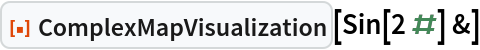Out=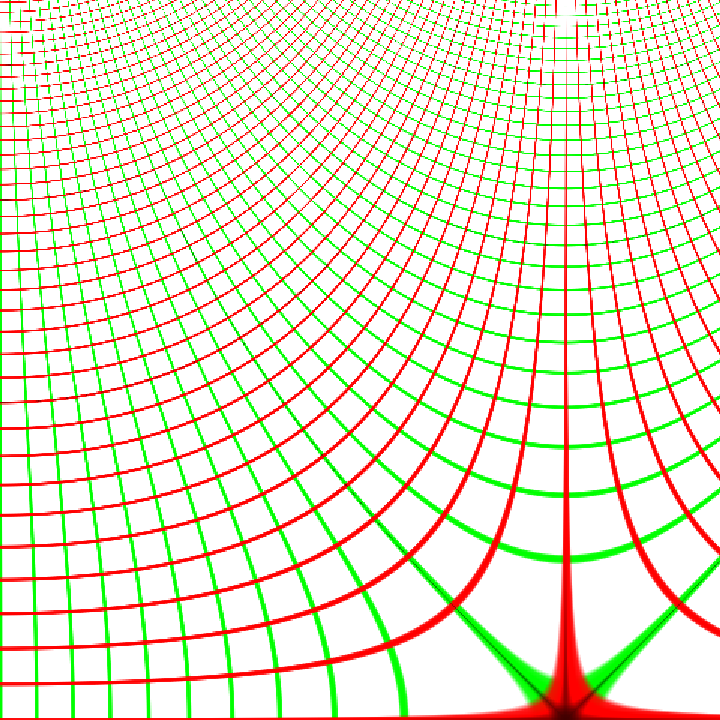Mappings on different sections of the complex plane can be visualized by specifying a different DataRange or PlotRange:

 In:=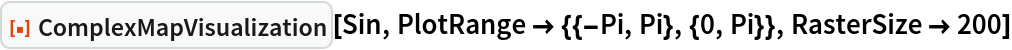Out=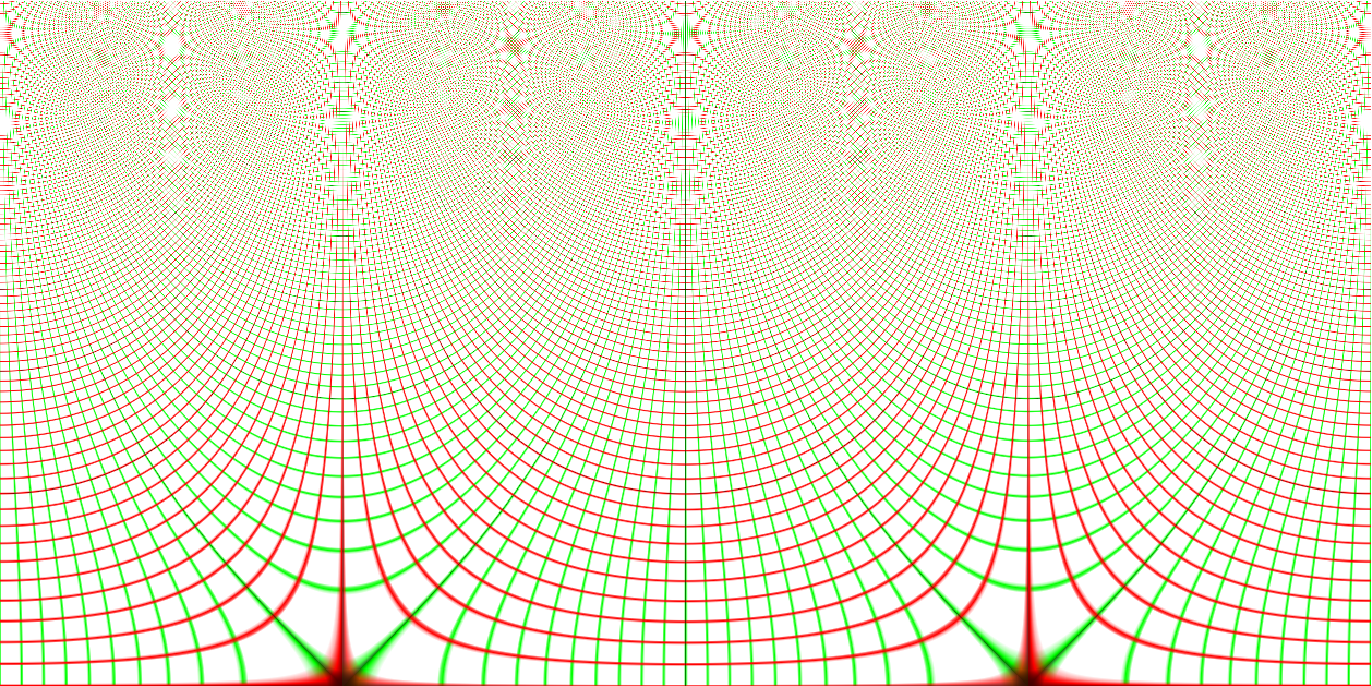Forward transformations can be created by using the inverse function:

 In:=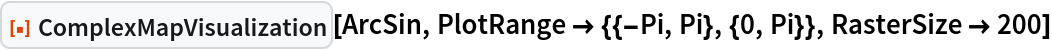Out=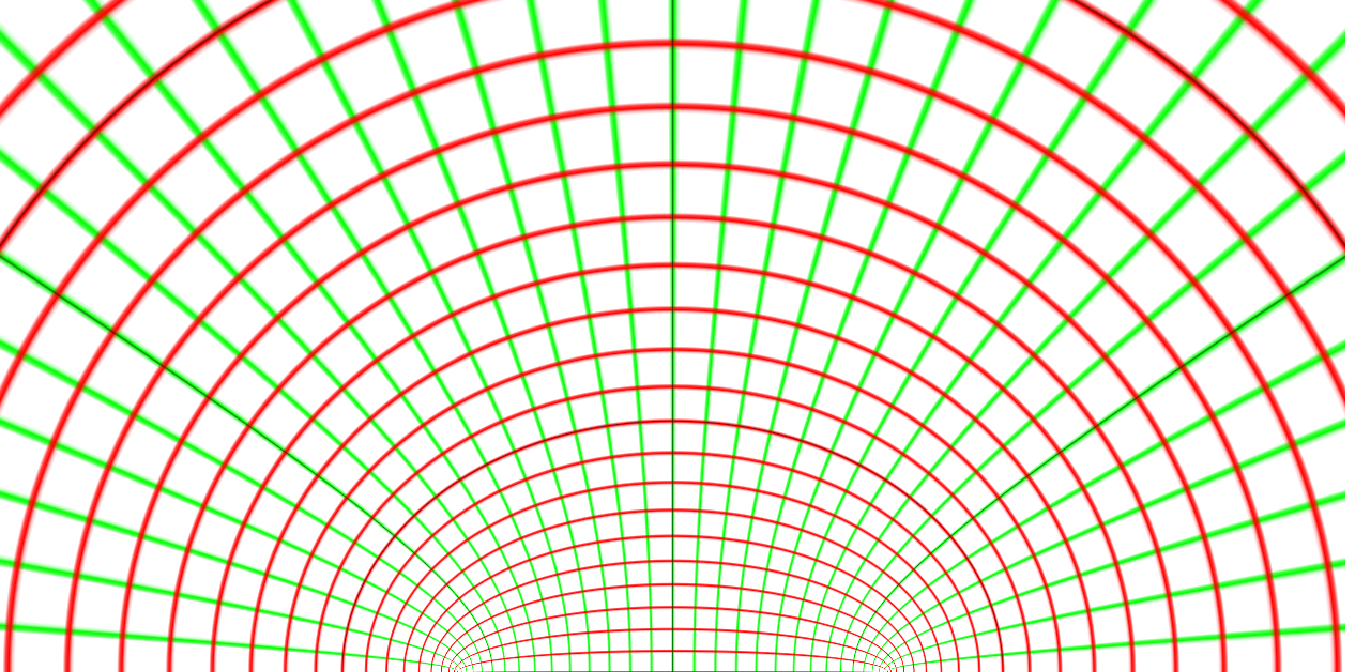### Scope (3)

Standard special functions can be visualized:

 In:=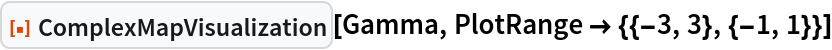Out=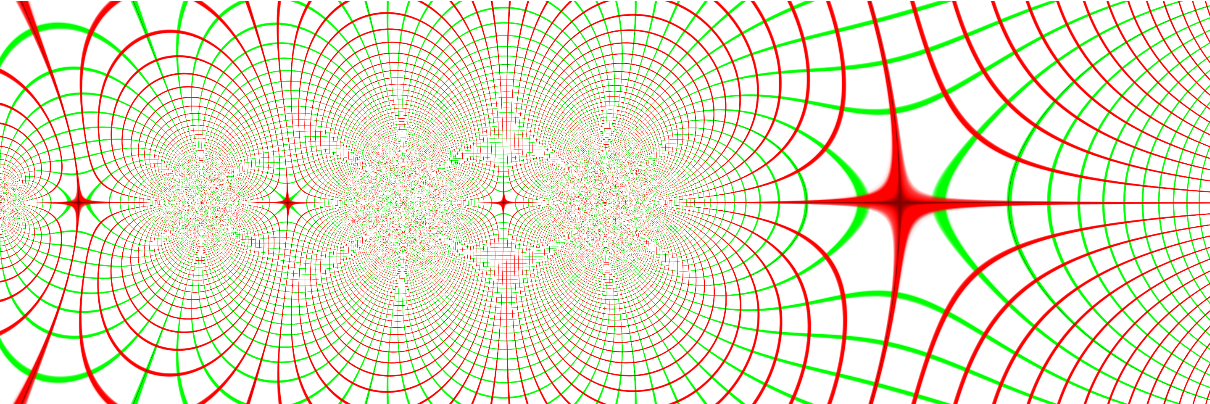General functions that have complex values as their domain and range can be visualized:

 In:=Out=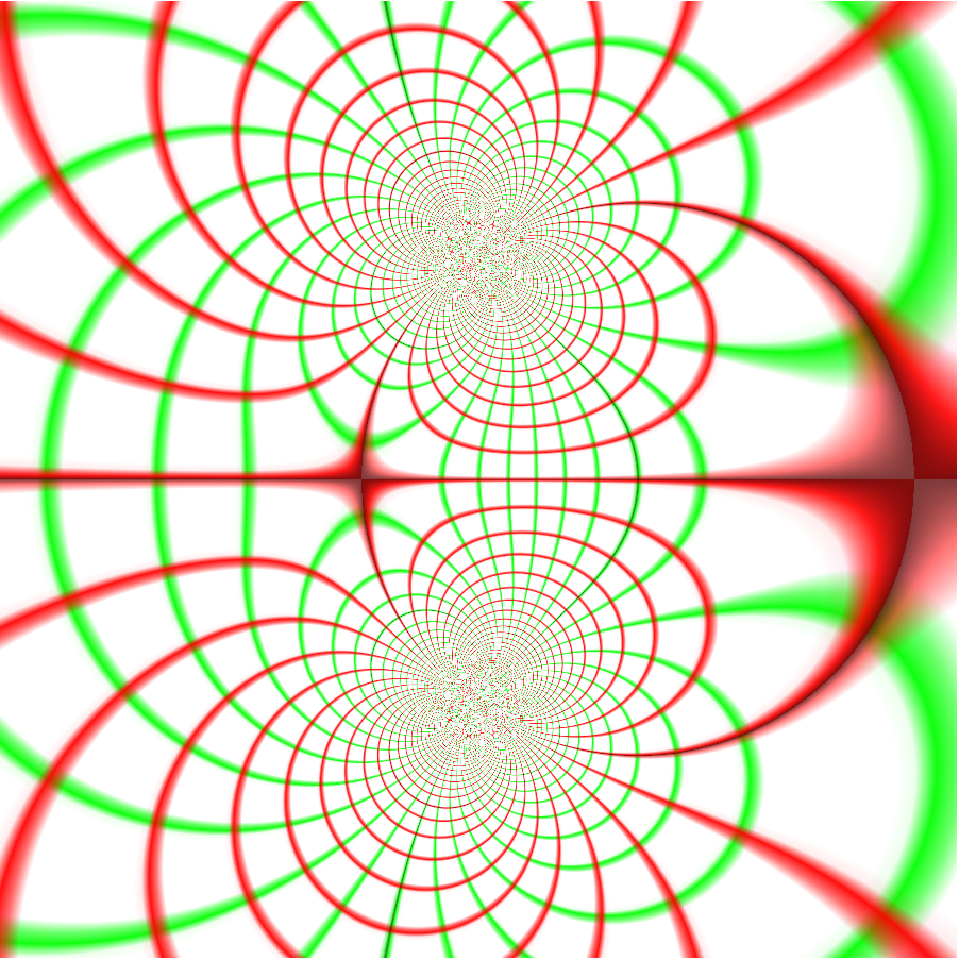Compiled functions can be visualized:

 In:=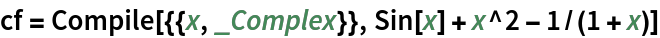Out=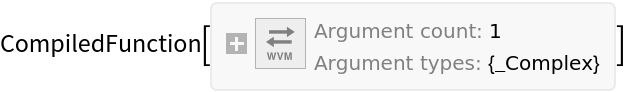In:=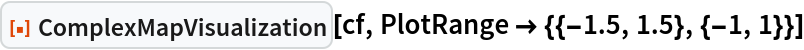Out=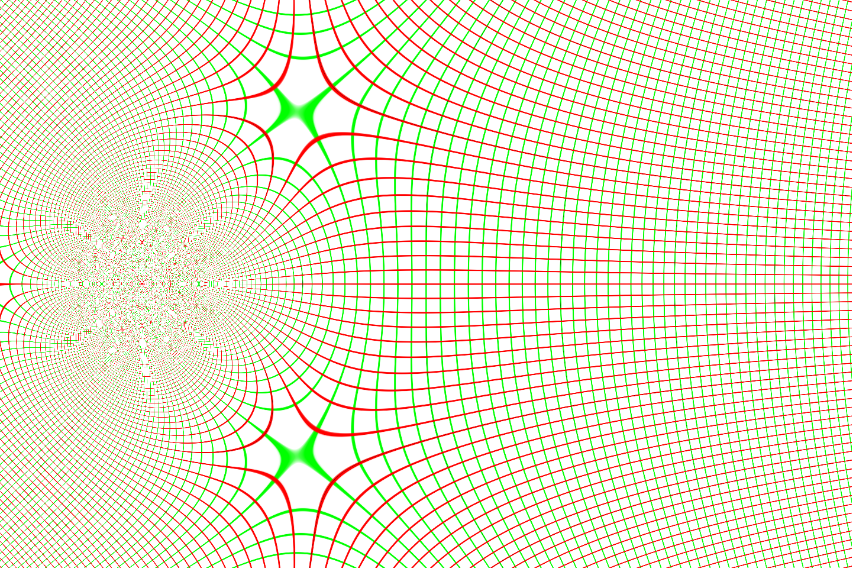### Options (2)

The "Image" option can be set to visualize mappings on arbitrary images or graphics objects:

 In:=Out=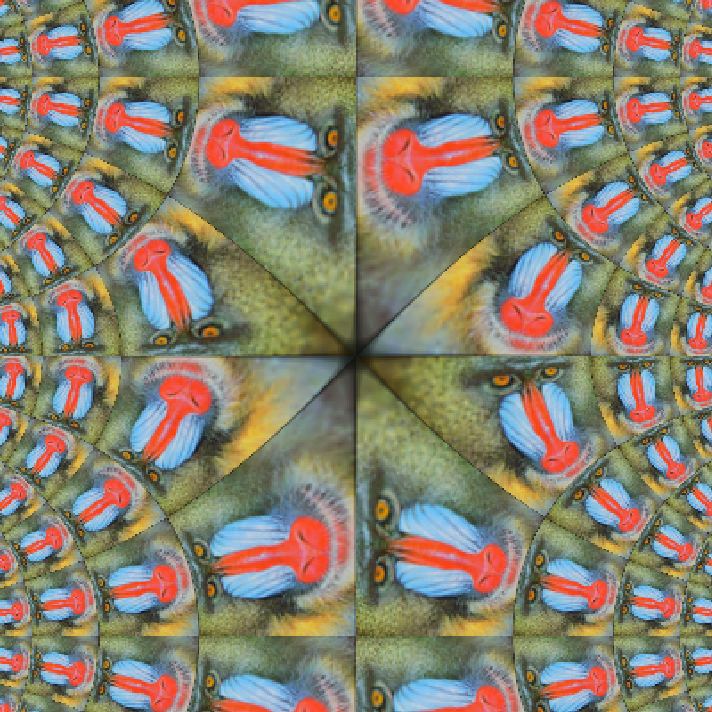Use the "Image" option to see the transformation of a polar grid:

 In:=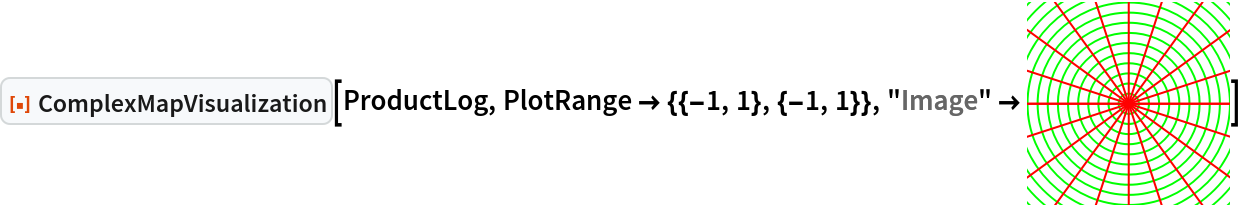Out=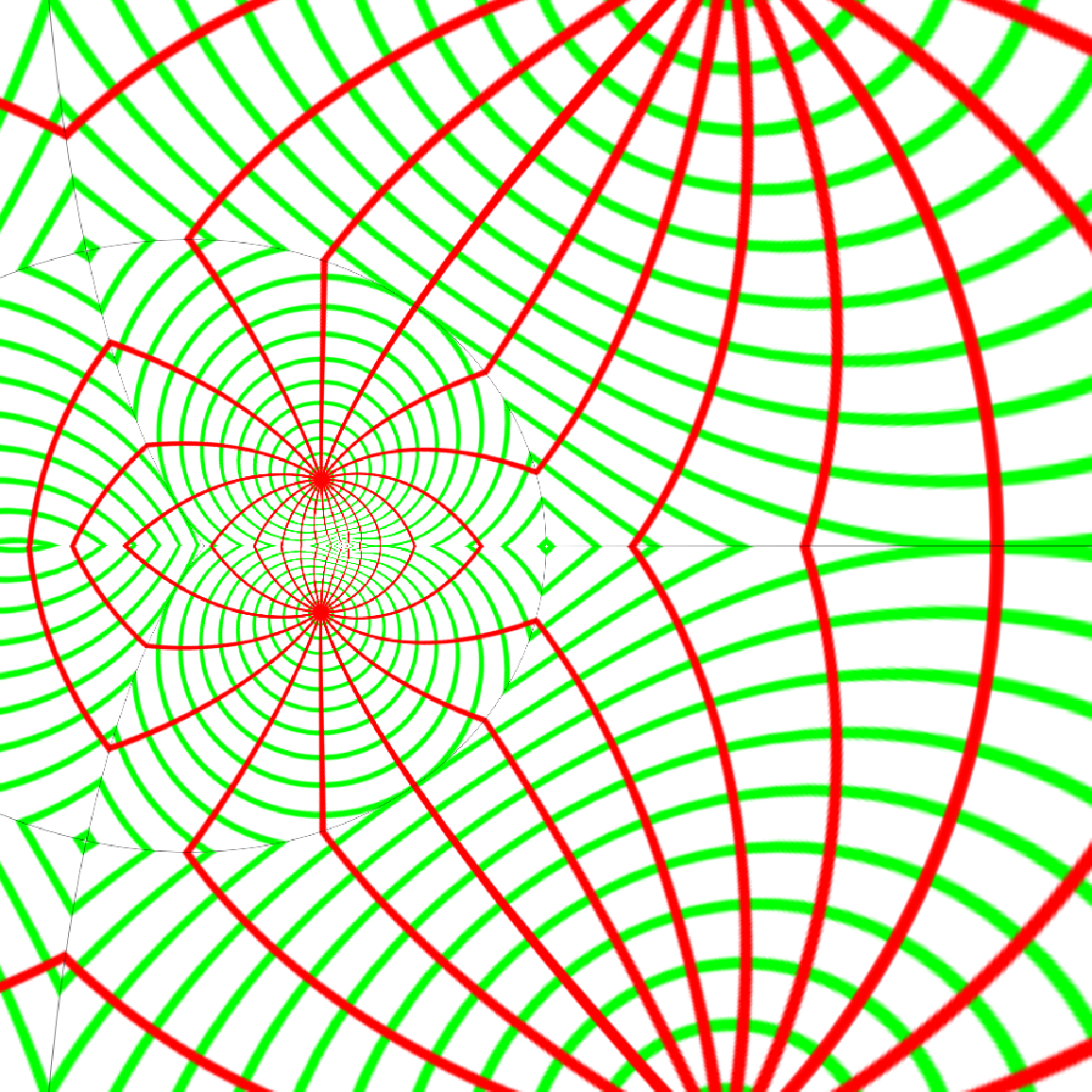Options available to Rasterize affect the quality of the resulting image:

 In:=Out=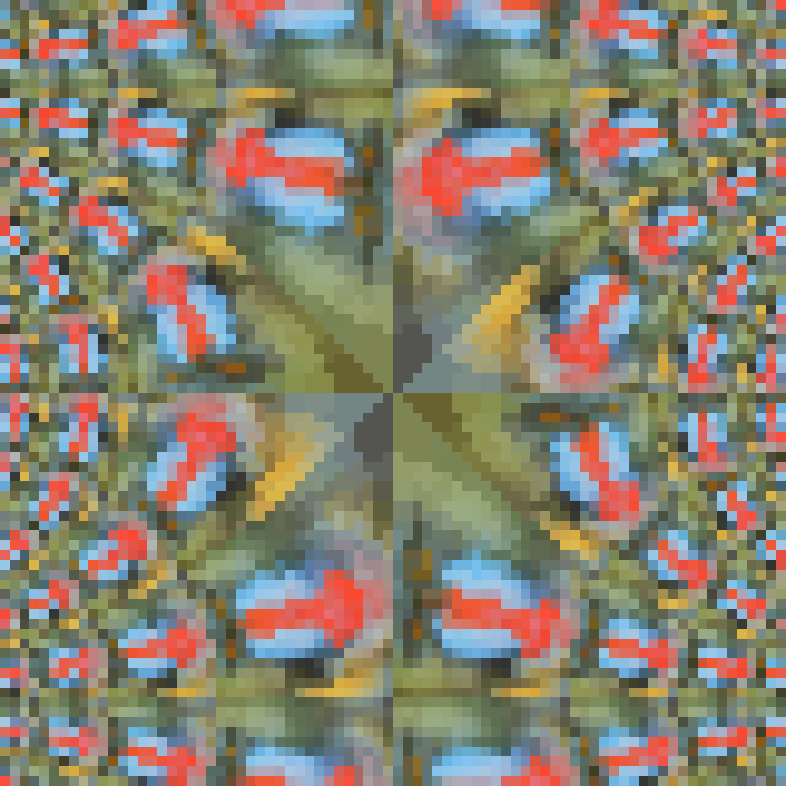### Properties and Relations (1)

Using ParametricPlot on the inverse function gives a result similar to the one produced by ComplexMapVisualization:

 In:=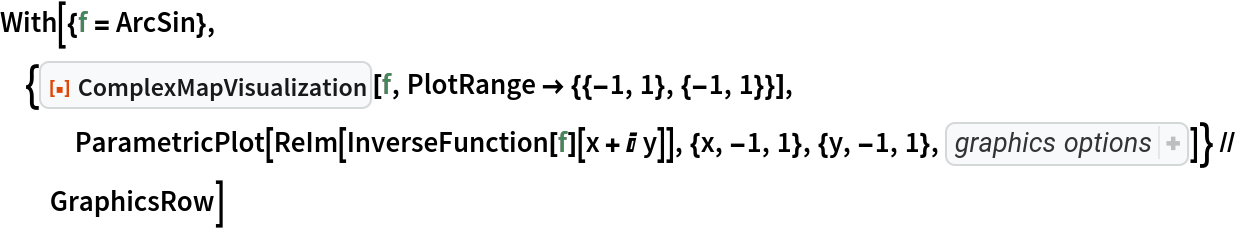Out=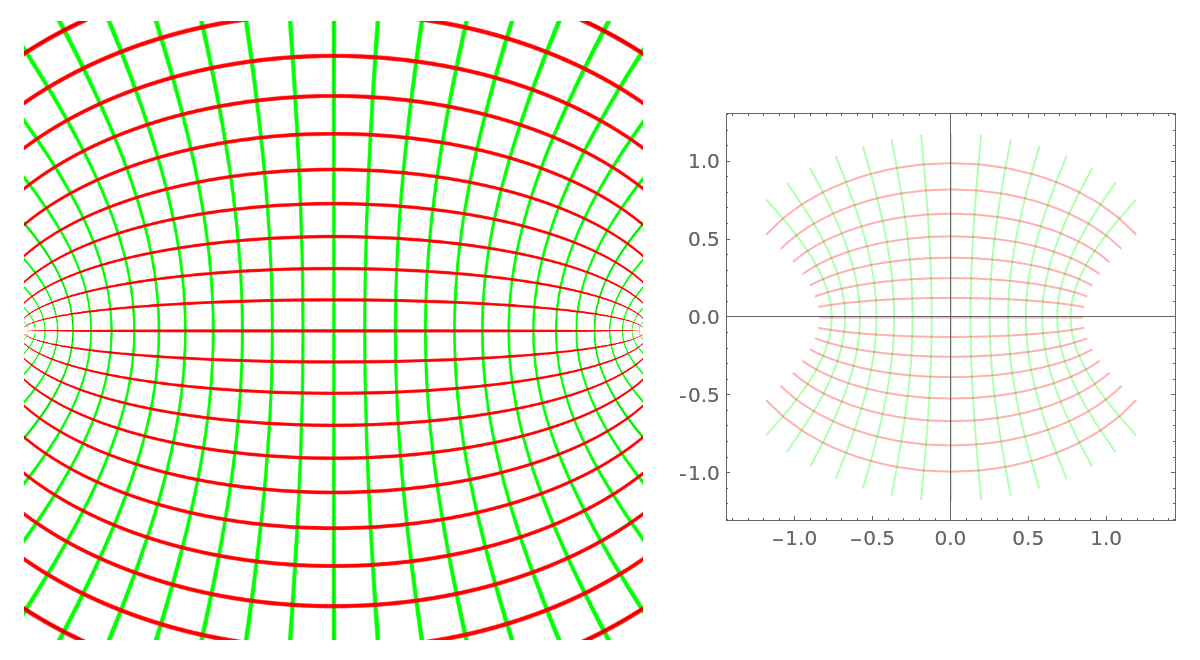### Possible Issues (2)

With a sufficiently large PlotRange, the computation may take a long time:

 In:=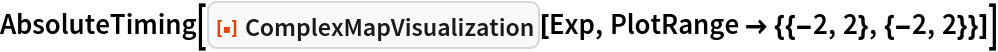Out=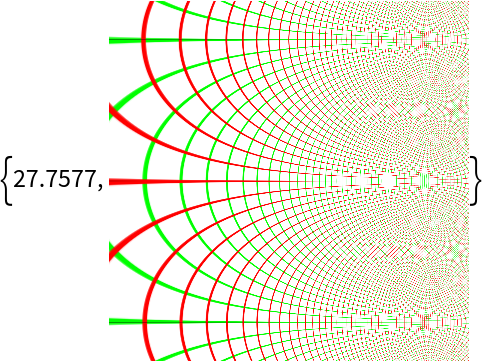You can use the RasterSize option to reduce the quality of the input image and reduce the computation time:

 In:=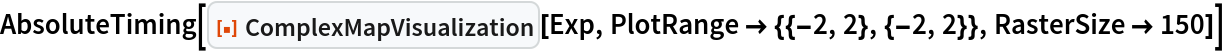Out=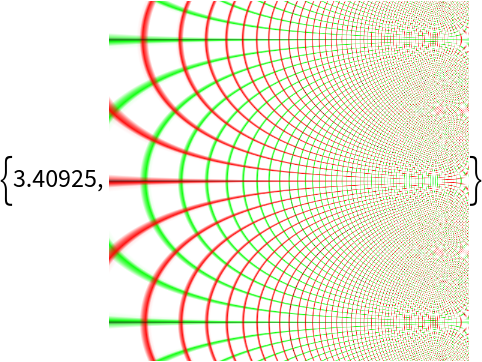## Publisher

Duncan Pettengill

## Version History

• 1.0.0 – 27 June 2022

## Author Notes

Credit to Oleg Marichev for highlighting the need for a good complex visualization function.